Hostname: page-component-797576ffbb-tx785 Total loading time: 0 Render date: 2023-12-02T06:37:13.506Z Has data issue: false Feature Flags: { "corePageComponentGetUserInfoFromSharedSession": true, "coreDisableEcommerce": false, "useRatesEcommerce": true } hasContentIssue false

# UNIQUENESS OF SOLUTIONS TO SCHRÖDINGER EQUATIONS ON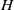$H$-TYPE GROUPS

Published online by Cambridge University Press:  07 August 2013

## Abstract

Core share and HTML view are not possible as this article does not have html content. However, as you have access to this content, a full PDF is available via the ‘Save PDF’ action button.

This paper deals with the Schrödinger equation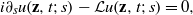$i{\partial }_{s} u(\mathbf{z} , t; s)- \mathcal{L} u(\mathbf{z} , t; s)= 0,$ where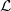$\mathcal{L}$ is the sub-Laplacian on the Heisenberg group. Assume that the initial data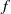$f$ satisfies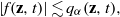$\vert f(\mathbf{z} , t)\vert \lesssim {q}_{\alpha } (\mathbf{z} , t),$ where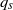${q}_{s}$ is the heat kernel associated to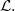$\mathcal{L} .$ If in addition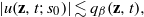$\vert u(\mathbf{z} , t; {s}_{0} )\vert \lesssim {q}_{\beta } (\mathbf{z} , t),$ for some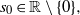${s}_{0} \in \mathbb{R} \setminus \{ 0\} ,$ then we prove that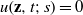$u(\mathbf{z} , t; s)= 0$ for all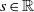$s\in \mathbb{R}$ whenever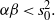$\alpha \beta \lt { s}_{0}^{2} .$ This result holds true in the more general context of$H$-type groups. We also prove an analogous result for the Grushin operator on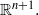${ \mathbb{R} }^{n+ 1} .$

## MSC classification

Type
Research Article
Information
Journal of the Australian Mathematical Society , December 2013 , pp. 297 - 314

## References

Berndt, J., Tricerri, F. and Vanhecke, L., Generalized Heisenberg Groups and Damek–Ricci Harmonic Spaces, Lecture Notes in Mathematics, 1598 (Springer, Berlin, 1995).Google Scholar
Chanillo, S., ‘Uniqueness of solutions to Schrödinger equations on complex semi-simple Lie groups’, Proc. Indian Acad. Sci. Math. Sci. 117 (2007), 325331.Google Scholar
Cowling, M., Escauriaza, L., Kenig, C. E., Ponce, G. and Vega, L., ‘The Hardy uncertainty principle revisted’, Indiana Univ. Math. J. 59 (6) (2010), 20072026.Google Scholar
Escauriaza, L., Kenig, C. E., Ponce, G. and Vega, L., ‘Hardy’s uncertainty principle, convexity and Schrödinger evolutions’, J. Eur. Math. Soc. (JEMS) 10 (2008), 883907.Google Scholar
Escauriaza, L., Kenig, C. E., Ponce, G. and Vega, L., ‘Convexity of free solutions of Schrödinger equations with Gaussian decay’, Math. Res. Lett. 15 (2008), 957971.Google Scholar
Escauriaza, L., Kenig, C. E., Ponce, G. and Vega, L., ‘The sharp Hardy uncertainty principle for Schrödinger evolutions’, Duke Math. J. 155 (2010), 163187.Google Scholar
Escauriaza, L., Kenig, C. E., Ponce, G. and Vega, L., ‘Uncertainty principles of Morgan type and Schrödinger evolutions’, J. Lond. Math. Soc. (2) 83 (2011), 187207.Google Scholar
Ionescu, I. D. and Kenig, C. E., ‘Uniqueness properties of solutions of Schrödinger equations’, J. Funct. Anal. 232 (2006), 90136.Google Scholar
Kaplan, A., ‘Fundamental solutions for a class of hypoelliptic PDE generated by composition of quadratic forms’, Trans. Amer. Math. Soc. 258 (1980), 147153.Google Scholar
Kenig, C. E., Ponce, G. and Vega, L., ‘On the support of solutions of nonlinear Schrödinger equations’, Comm. Pure Appl. Math. 56 (2002), 12471262.Google Scholar
Ludwig, J. and Müller, D., ‘Uniqueness of solutions to Schrödinger equations on 2-step nilpotent Lie groups’, 2012, arXiv:1207.4652.Google Scholar
Pasquale, A. and Sundari, M., ‘Uncertainty principles for the Schrödinger equation on Riemannian symmetric spaces of the noncompact type’, Ann. Inst. Fourier (Grenoble) 62 (2012), 859886.Google Scholar
Randall, J., ‘The heat kernel for generalized Heisenberg groups’, J. Geom. Anal. 6 (1996), 287316.Google Scholar
Ricci, F., ‘Commutative algebras of invariant functions on groups of Heisenberg type’, J. Lond. Math. Soc. 32 (1985), 265271.Google Scholar
Robbiano, L., ‘Unicité forte à l’infini pour KdV’, ESAIM Control Optim. Calc. Var. 8 (2002), 933939.Google Scholar
Thangavelu, S., Lectures on Hermite and Laguerre expansions, Mathematical Notes, 42 (Princeton University Press, Princeton, 1993).Google Scholar
Thangavelu, S., An Introduction to the Uncertainty Principle. Hardy’s Theorem on Lie Groups, Progress in Mathematics, 217 (Birkhäuser, Boston, MA, 2004).Google Scholar
Tuan, V.-K., ‘Uncertainty principles for the Hankel transform’, Integral Transforms Spec. Funct. 18 (2007), 369381.Google Scholar
Zhang, B.-Y., ‘Unique continuation for the Korteweg-de Vries equation’, SIAM J. Math. Anal. 23 (1992), 5571.Google Scholar